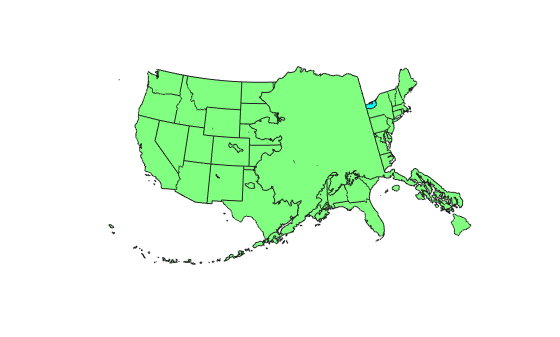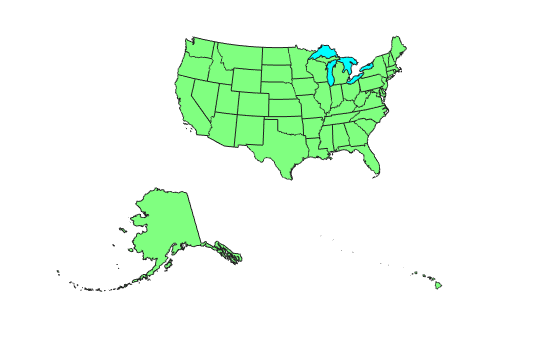# axesscale

Resize axes for equivalent scale

## Syntax

``axesscale``
``axesscale(hbase)``
``axesscale(hbase,hother)``

## Description

````axesscale` resizes all axes in the current figure to have the same scale as the current axes (`gca`). In this context, scale means the relationship between axes x- and y-coordinates and figure and paper coordinates. When `axesscale` is used, a unit of length in x and y is printed and displayed at the same size in all the affected axes. The `XLimMode` and `YLimMode` of the axes are set to `'manual'` to prevent autoscaling from changing the scale.```

example

````axesscale(hbase)` uses the axes `hbase` as the reference axes, and rescales the other axes in the current figure.```
````axesscale(hbase,hother)` uses the axes `hbase` as the base axes, and rescales only the axes in `hother`.```

## Examples

collapse all

Display the conterminous United States, Alaska, and Hawaii in separate axes in the same figure, with a common scale.

```states = shaperead('usastatehi', 'UseGeoCoords', true); statenames = {states.Name}; alaska = states(strcmp('Alaska', statenames)); hawaii = states(strcmp('Hawaii', statenames));```

Create a figure for the conterminous states.

```f = figure; hconus = usamap('conus'); geoshow(states, 'FaceColor', [0.5 1 0.5]); load conus gtlakelat gtlakelon geoshow(gtlakelat, gtlakelon,... 'DisplayType', 'polygon', 'FaceColor', 'cyan') framem off; gridm off; mlabel off; plabel off```Display Alaska and Hawaii on different axes. For now, the axes overlap.

```halaska = axes('Parent',f); usamap('alaska') geoshow(alaska, 'FaceColor', [0.5 1 0.5]); framem off; gridm off; mlabel off; plabel off hhawaii = axes('Parent',f); usamap('hawaii') geoshow(hawaii, 'FaceColor', [0.5 1 0.5]); framem off; gridm off; mlabel off; plabel off```Arrange the axes so they do not overlap. However, this changes the scale of the axes.

```set(hconus, 'Position',[0.1 0.35 0.85 0.6]) set(halaska,'Position',[0.02 0.08 0.2 0.2]) set(hhawaii,'Position',[0.5 0.1 0.2 0.2])```Resize the Alaska and Hawaii axes based on the size of the conterminous United States.

`axesscale(hconus)`## Limitations

The equivalence of scales holds only as long as no commands are issued that can change the scale of one of the axes. For example, changing the units of the ellipsoid or the scale factor in one of the axes would change the scale.

## Tips

To ensure the same map scale between axes, use the same ellipsoid and scale factors.